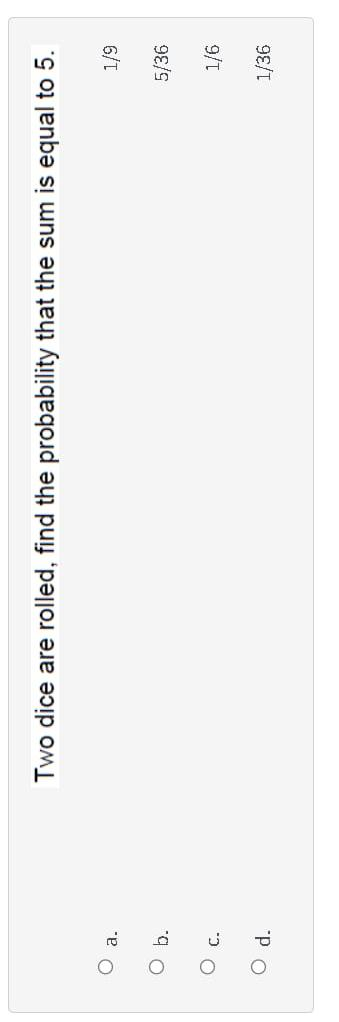Home / Expert Answers / Statistics and Probability / two-dice-are-rolled-find-the-probability-that-the-sum-is-equal-to-5-a-1-9-b-5-36-pa129

# (Solved): Two dice are rolled, find the probability that the sum is equal to 5 . a. \( 1 / 9 \) b. \( 5 / 36 ...Two dice are rolled, find the probability that the sum is equal to 5 . a. \( 1 / 9 \) b. \( 5 / 36 \) c. \( 1 / 6 \) d. \( 1 / 36 \)

We have an Answer from Expert